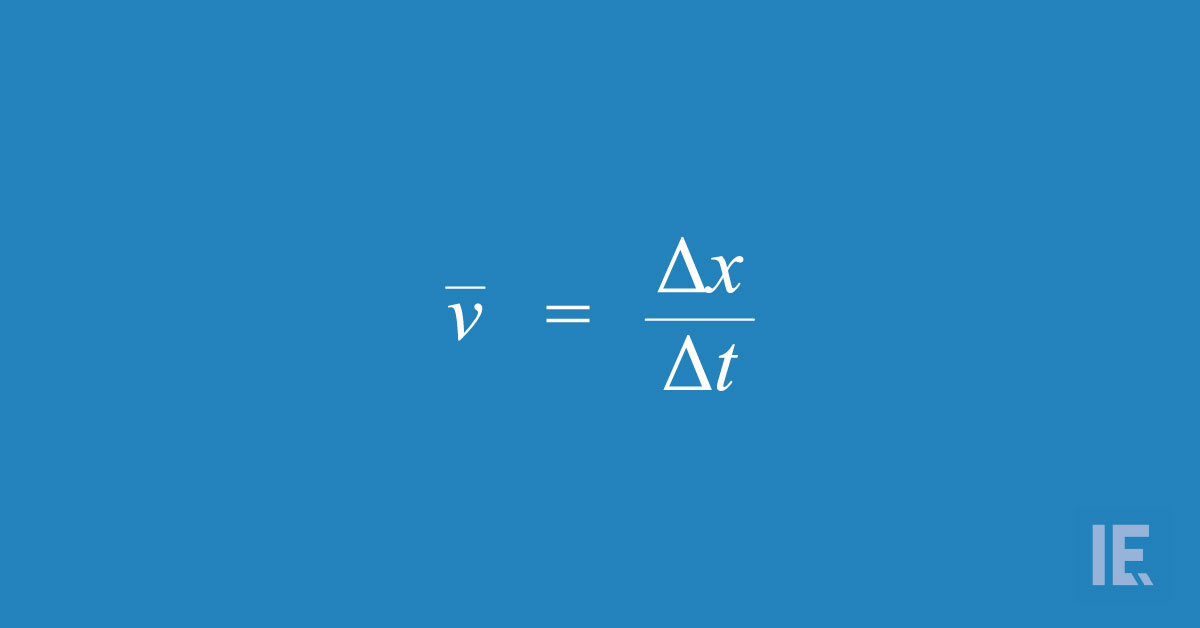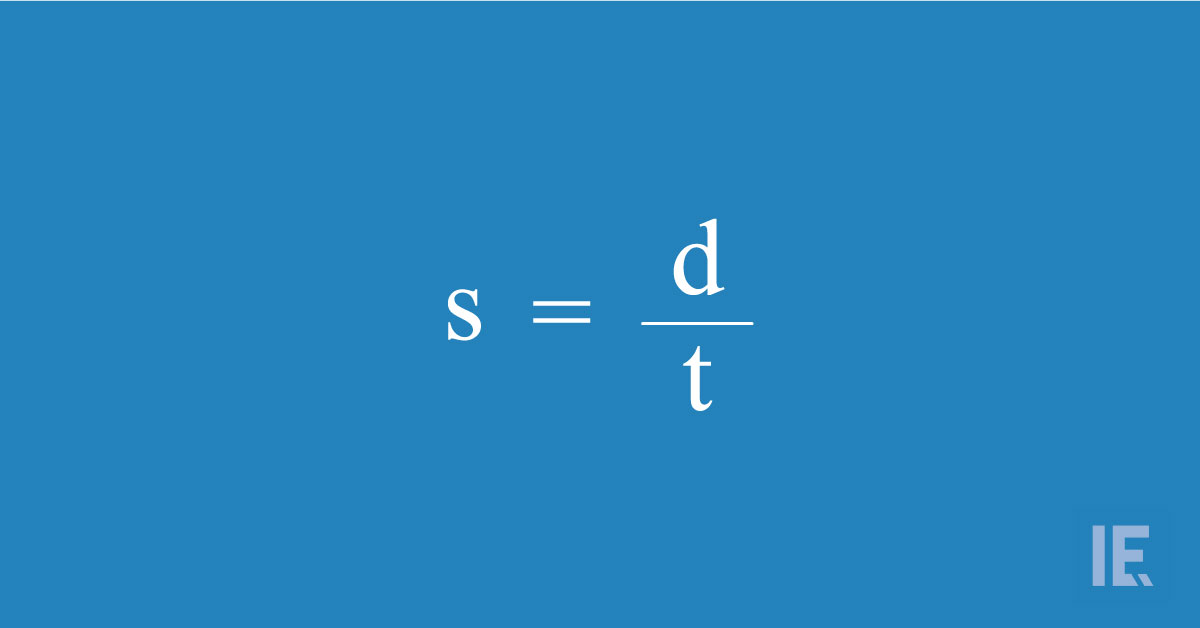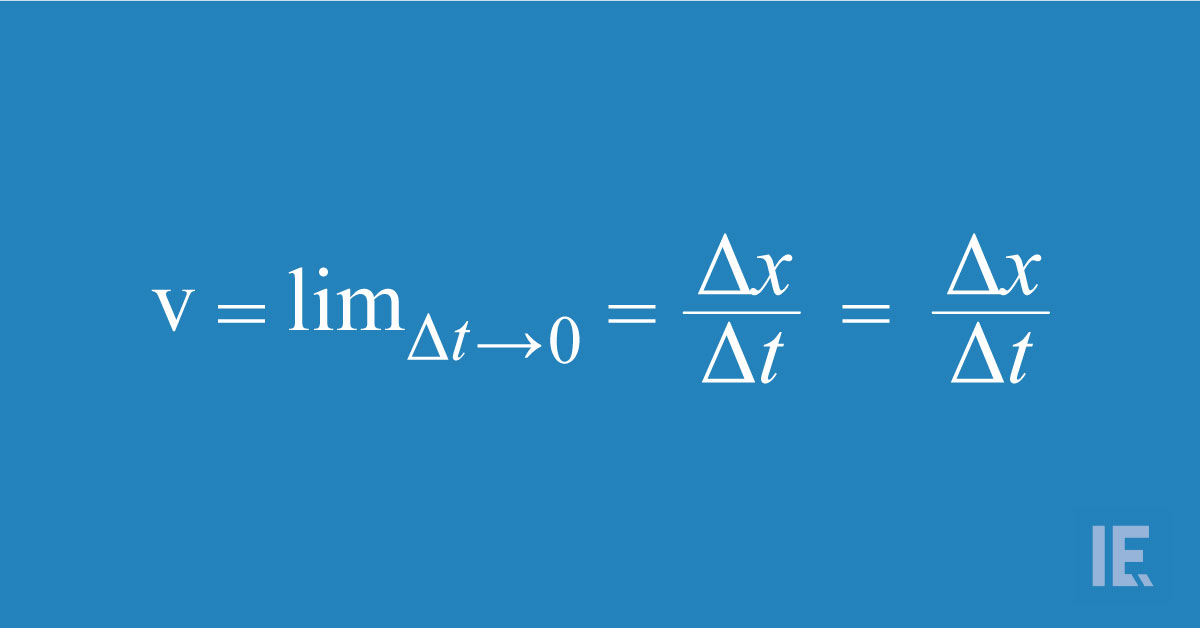# Speed Vs Velocity: Understanding the Difference

What makes velocity different from speed?Velocity is the rate of change of motion in a specific direction. The definition seems very simple, yet this vector quantity is complex in more ways than one.

The equation of average velocity is as follows:Where,

Δx = position or displacement
Δt = Time interval

The line or sometimes an arrow over ν signifies that this value is a vector.

RELATED: UNDERSTANDING THE PHYSICS OF FIDGET SPINNERS

According to the equation, the value of velocity increases as the time required for displacement decreases. So, we say high velocity when the time required to cross a distance is low.

Likewise, low velocity is a result of more time required to make a displacement.

## Displacement or distance

You might have noticed that we use the term displacement with velocity rather than distance. There is a reason why we chose to do so!

Distance is a scalar quantity and is a measure of the magnitude of distance covered. It is just a number that specifies how much an object has traveled from point A to point B.

Now imagine two points, separated by a distance. There are infinite ways to connect them.

The shortest distance would be a single line connecting these two points.

However, you can also connect two points using curved lines. But the distance will be different for every type of line you choose to connect the two points.

We cannot say the same for displacement because it is the measure of how far the second point is from the reference point.

Therefore, displacement has both magnitude and direction, making it a vector quality. When defining velocity, you are defining not just the rate of motion, but also its direction.

## Speed vs Velocity

This is another comparison that leaves many scratching their heads. People often use the term velocity and speed interchangeably, but they both have very distinct qualities.

Let us examine the equation of speed.There’s a striking similarity between the equation of speed and velocity. The equation tells us that speed is the rate of distance covered in a specific time frame.

But here, we are concerned only about the distance. Hence, speed is a scalar quantity that gives us the rate of motion without any information about the direction.

However, when we re-examine velocity, we can see that distance is replaced by displacement. This gives us an idea about the direction of the object.

Therefore, the velocity and speed of an object are both defined differently.

For example, let us imagine that a car travels at 20kmph. If you ask a physicist, he/she would say that the speed of the car is 20kmph but the velocity is unknown.

But if you know that the car is traveling at 20kmph to the North, then the physicist would say that the speed of the car is 20kmph and its velocity is 20kmph in the North direction.

## Can the speed and velocity of an object be different?

There is always a comparison drawn between speed and velocity regarding the motion of an object. These two entities are constantly pitted against each other to see which is superior.

But there is no point comparing vector and scalar quantities because they are distinct in their ways.

To give a rough idea, consider an object traveling in a perfect circle at 20 kmph. Since this is a perfect circle and we have defined a constant rate of motion for it, speed and velocity should be the same, right?

Wrong! In this case, the speed of the object is constant, but the velocity is always changing.

This is because we consider direction when defining velocity. So when an object travels in a circle, we find that its direction is always in a state of change.

If we find out the direction of that object at any given point, it will be tangential to the outline edge of that circle.

## Instantaneous Velocity and Instantaneous Speed

When we talk about the standard equation, almost always, we are talking about average speed and average velocity. However, sometimes it is necessary to know the velocity or speed at an instant.

The velocity of an object at a specific point in time is known as instantaneous velocity. The general equation for instantaneous velocity is:The speed of an object at a specific point in time is known as instantaneous speed. The general equation for instantaneous speed is:Where,
x = Displacement
s = Distance

The most common example of the measurement of instantaneous speed is a speedometer. Speedometers show the speed of an object in a particular instant, not its average value.

## Why is velocity important?

Why bother having two equations for the rate of motion with respect to the time when you can simply use speed, which has a simpler equation?

The reason why velocity is of high importance is that, in physics, the direction of an object carries great importance.

Consider an airplane en route to its destination. To get detailed information on the airplane, air control terminals use velocity instead of the speed.

Vectors also play a very important role in helping us determine how the velocity of one object may influence another object. For example, if two objects collide in a nonlinear fashion, understanding their velocity before impact will help us understand their behavior (direction and speed) after impact.

This is not possible if you are using only the speed of the two objects. There are many more examples that you can draw when it comes to the importance of velocity in physics.

RELATED: THE MOST MISUNDERSTOOD THEORIES IN PHYSICS - EXPLAINED

## Conclusion

Velocity often gets overlooked because it doesn’t serve major roles outside of physics. However, within the realm of physics, it takes the center stage while speed is regarded with a lesser importance.

Irrespective of how important both these quantities are, understanding the differences between velocity and speed gives us great insight into the different aspects of science and its applications.SHOW COMMENT (1)Job Board